# SBI Clerk Mains Reasoning Ability Quiz – 1

## SBI Clerk Mains Reasoning Ability Quiz

Directions (1-5): Study the information carefully and answer the questions given below.

In a certain code language,

“Wrong message being sent” is written as ‘L7  ZVV9  VR7  V6′

“Actors copying idiots” is written as ‘ZL8  RL9  RRL8 ’

“People split personality” is written as ‘VVL8  R7  ZVRL13’

1. Which of the following represents the code for ‘notication’?

(a) ZRRLO14

(b) RRRRZL14

(c) RRRZZL14

(d) ZRRRLL14

(e) None of these.1. Which of the following will be the code for ‘Toxicity’?

(a) RLF11

(b) ROE11

(c) RLP11

(d) RRL10

(e) RRY11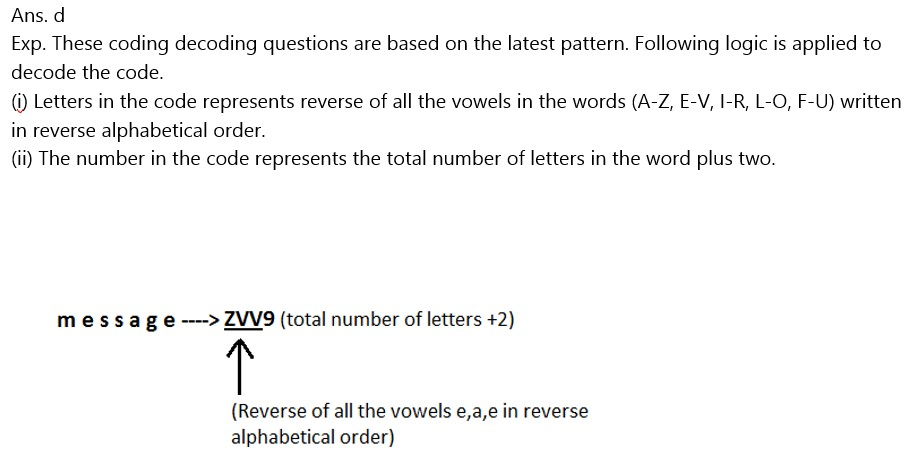1. Which of the following is the code for ‘Indian’?

(a) ZRR8

(b) RZZ8

(c) IRZ7

(d) ZRR6

(e) None of these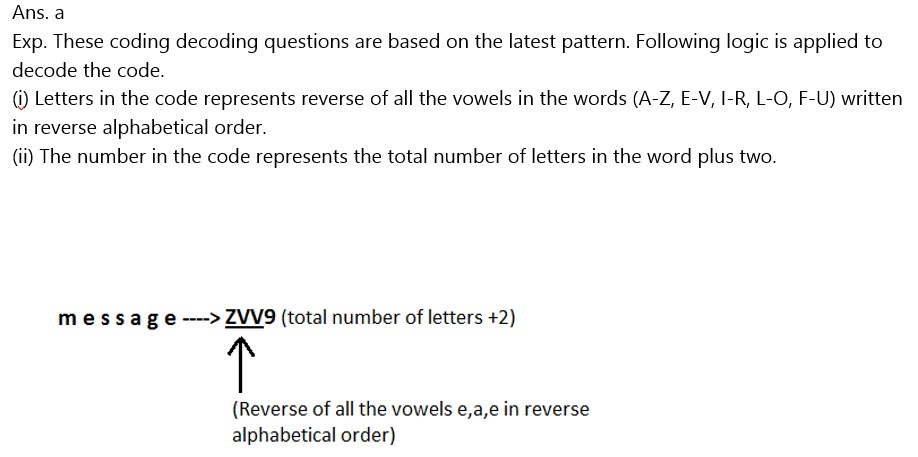1. Which of the following could be coded as ‘ZL10’?

(a) copycats

(b) floppydisk

(c) computer

(d) continue

(e) None of these1. Which of the following words could be coded as ‘VRL9’?

(a) Sharks

(b) Kings

(c) Giants

(d) Pheonix

(e) None of these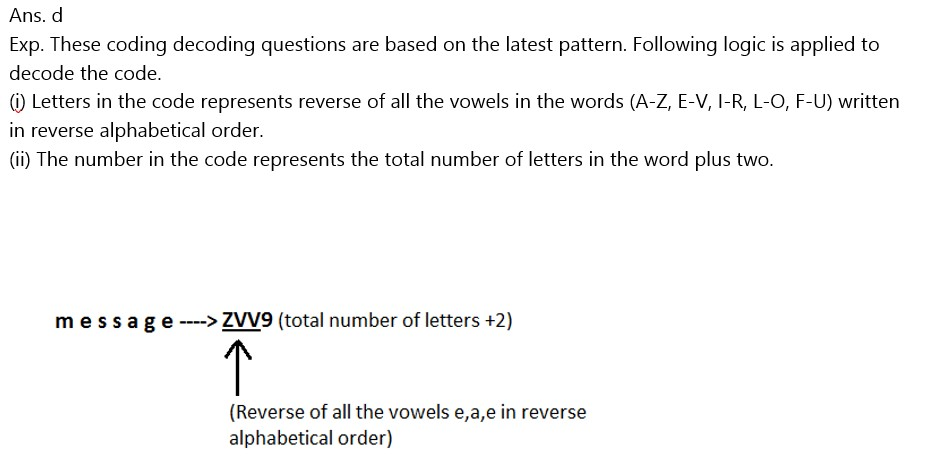Directions (6-8): Read the following information carefully and answer the questions that follows:

Seven children are standing in a park at seven different points A, B, C, D, P, Q and R.

(i) B is 3m to the south of A and 4m to the west of C.

(ii) Shortest distance between P and R is 3m.

(iii) Angle BCD = 90°.

(iv) Shortest distance between P and Q is 3m.

(v) Points B, C and P are in a straight line. Similarly, points D, Q and P are also in straight line.

(vi) Point D is to the south-west of P.

(vii) Shortest distance between C and D is 4m.

(viii) Shortest distance between Q and R is 3m.

(xi) Shortest distance between Q and D is 2m.

1. What is the shortest distance between C and P?

(a) 4m

(b) 5m

(c) 2m

(d) 3m

(e) Cannot be determined1. How many childrens are facing North?

(a) Three

(b) More than three

(c) Two

(d) None of these

(e) Cannot be determined1. How many children’s are standing to the west of P?

(a) None

(b) Two

(c) One

(d) Three

(e) None of these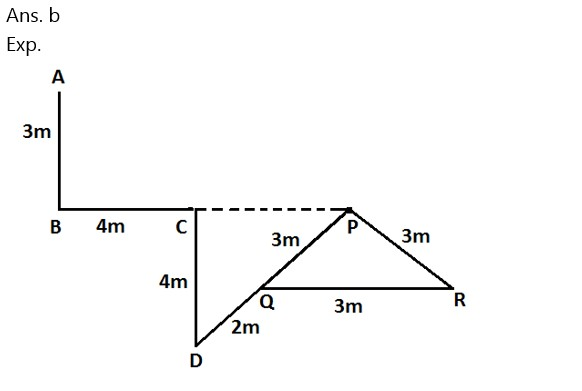Directions (9-10): In these questions, relationship between different elements is. shown in the statements. The statements are followed by conclusions. Study the conclusions based on the given statements and select the appropriate answer.

1. Statement: M D > E < C = N, F E < L

Conclusions : I. L > N   II. M N

(a) Only conclusion II is true

(b) Either conclusion I or II is true

(c) Both conclusions I and II are true

(d) Neither conclusion I nor II is true

(e) Only conclusion I is true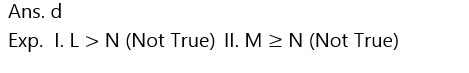1. Statements: A B < E F, E = D < N M

Conclusions: I. A < N    II. N > F

(a) Both conclusions I and II are true

(b) Only conclusion I is true

(c) Neither conclusion I nor II is true

(d) Either conclusion I or II is true

(e) Only conclusion II is true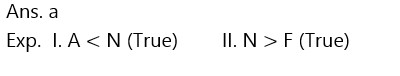### 2022 Preparation Kit PDF

#### Most important PDF’s for Bank, SSC, Railway and Other Government Exam : Download PDF Now

AATMA-NIRBHAR Series- Static GK/Awareness Practice Ebook PDF Get PDF here
The Banking Awareness 500 MCQs E-book| Bilingual (Hindi + English) Get PDF here
AATMA-NIRBHAR Series- Banking Awareness Practice Ebook PDF Get PDF here
Computer Awareness Capsule 2.O Get PDF here
AATMA-NIRBHAR Series Quantitative Aptitude Topic-Wise PDF 2020 Get PDF here
Memory Based Puzzle E-book | 2016-19 Exams Covered Get PDF here
Caselet Data Interpretation 200 Questions Get PDF here
Puzzle & Seating Arrangement E-Book for BANK PO MAINS (Vol-1) Get PDF here
ARITHMETIC DATA INTERPRETATION 2.O E-book Get PDF here

3